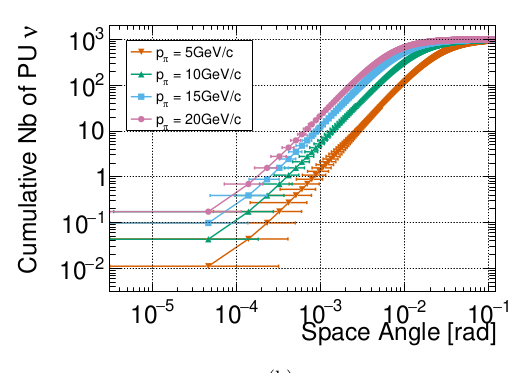Filled Area for TGraph Errors with Horizontal Error bars

Dear Root Experts,

I have a TGraph with 0 vertical error bars and non-0 horizontal error bars.
I would like to draw it with an filled area like the option “4” for vertical errors bars.
Do you see simple ways of doing it?
Thanks a lot

Mathieu

You need vertical error bars. option “4” needs them.

Thanks a lot!
Indeed, I understood that “4” works only for vertical error bar, but my error bars are horizontal. I cannot convert them everywhere to vertical, especially at the borders like on this plot:Any ideas?

Well, yes I can see a solution. You need to make new closed TGraph build with the end points of the errors bars:

{
c1 = new TCanvas("c","c",200,10,700,500);
const Int_t n = 20;
Double_t x[n], y[n], ex[n], ey[n];
for (Int_t i=0;i<n;i++) {
x[i]  = i*0.1;
y[i]  = 10*sin(x[i]+0.2);
ex[i] = 0.08;
ey[i] = 0.0;
}
auto *gr = new TGraphErrors(n,x,y,ex,ey);

auto *gr2 = new TGraph();
gr2->SetFillColor(2);
for (int i = 0;  i<20; i++) gr2->AddPoint(gr->GetPointX(i)+gr->GetErrorX(i),gr->GetPointY(i));
for (int j = 19; j>=0; j--) gr2->AddPoint(gr->GetPointX(j)-gr->GetErrorX(j),gr->GetPointY(j));
gr2->Draw("ALF");
gr->Draw("L");
}

Wonderful thanks a lotThis topic was automatically closed 14 days after the last reply. New replies are no longer allowed.